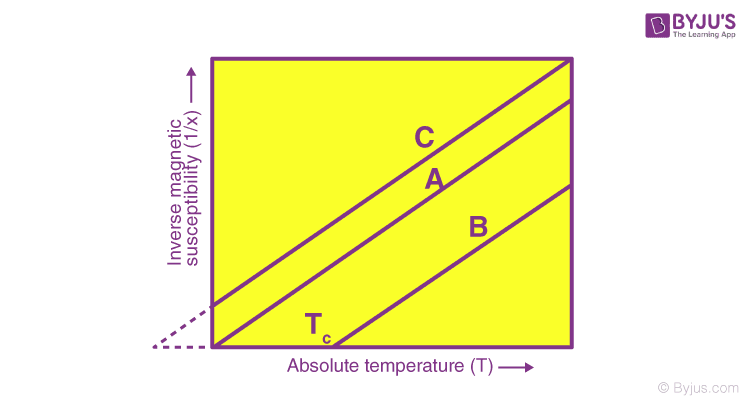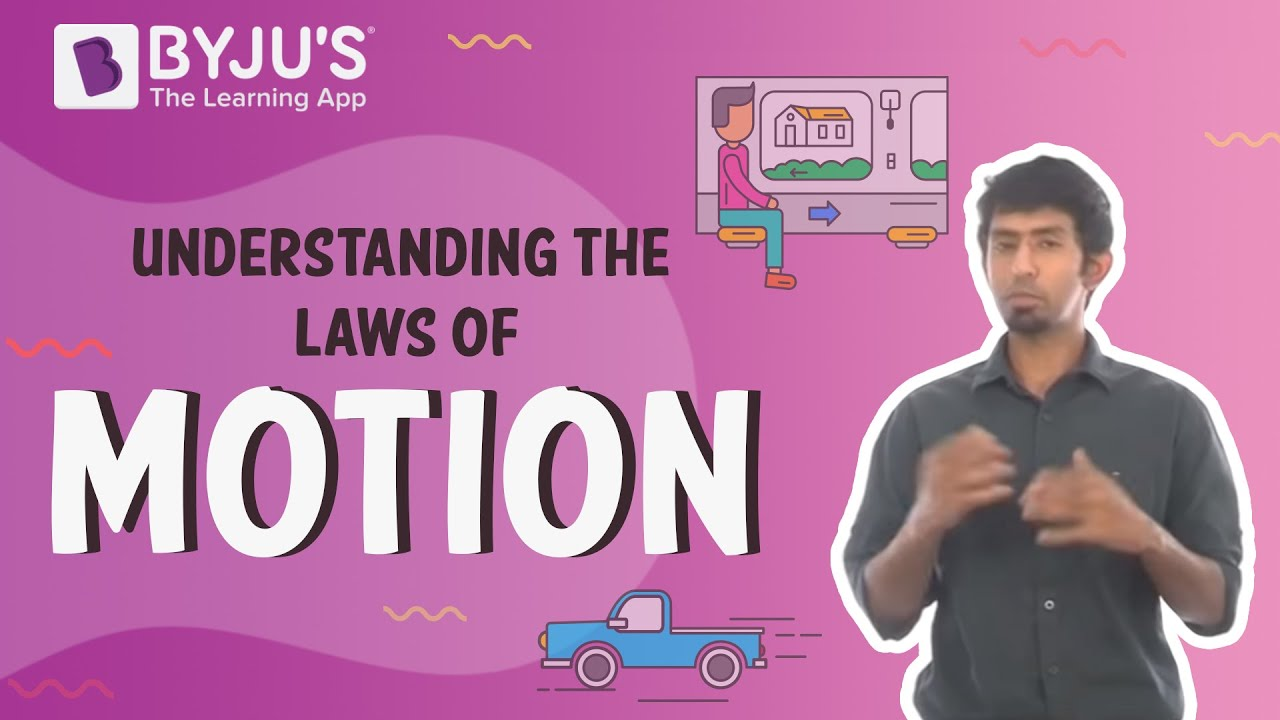Checkout JEE MAINS 2022 Question Paper Analysis : Checkout JEE MAINS 2022 Question Paper Analysis :

# Curie-Weiss Law

The Curie-Weiss law is one of the important laws in electromagnetism that says that the magnetic susceptibility is above the Curie temperature point of a ferromagnet in the paramagnetic region. The magnetic moment is a quantity of a magnet that determines its torque in an external magnetic field. Example: a bar magnet, electric current loop, a molecule and an electron all have a magnetic moment.The magnetic polarization or magnetization of a magnetic material expresses the density of induced or permanent magnetic moments in the vector field. The magnetic moment can develop from the microscopic electric current that is generated by the spin of the electrons or motion of electrons in an atom or the spin of the nuclei.

$$\begin{array}{l}X = \frac{C}{T – T_{c}}\end{array}$$

Here,

$$\begin{array}{l}C\end{array}$$
= Material specific Curie

$$\begin{array}{l}T\end{array}$$
= Absolute temperature

$$\begin{array}{l}{T_{c}}\end{array}$$
= Curie temperature

The net magnetization depends on the response of the external magnetic field’s materials. However, they may be even present in the absence of the external magnetic field, for example, in a cold iron as a spontaneous magnetization. Well, other materials that have similar properties are magnetite and nickel, these are called ferromagnets. The temperature in which a ferromagnetic material is called Curie temperature.

## Curie-Weiss Law Limitation

The Curie-Weiss law holds false in many materials to describe the susceptibility. Instead, there is a critical behavior of the form.

$$\begin{array}{l}X \sim \frac{1}{\left (T – T_{c} \right )^{\gamma}}\end{array}$$

At temperature

$$\begin{array}{l}T \gg T_{c}\end{array}$$
the expression of the law still holds true. But, with the
$$\begin{array}{l}T_{c}\end{array}$$
will be replaced by temperature
$$\begin{array}{l}\left (\theta \right )\end{array}$$
is higher than the Curie temperature.

Stay tuned with BYJU’S for more such interesting articles. Also, register to “BYJU’S – The Learning App” for loads of interactive, engaging Physics-related videos and an unlimited academic assist.

## Understand the Laws of Motion and the concepts behind these theories by watching this intriguing video.Test Your Knowledge On Curie Weiss Law!